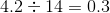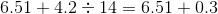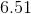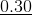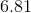# SSAT Middle Level Math : How to divide fractions

## Example Questions

← Previous 1 3 4 5 6 7 8 9 17 18

### Example Question #1 : How To Divide Fractions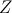is larger than 0.  Which of the following could be equal to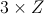?

I.II.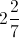III.I and II

III only

I only

II only

I, II and III

I, II and III

Explanation:

All of the answers can be divided by 3 to yield an answer larger than zero.  In fact, any positive number would be a viable answer.

### Example Question #2 : How To Divide Fractions

Express the quotient as a fraction in lowest terms: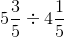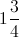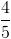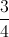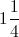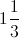Explanation:

Rewrite the mixed fractions as improper fractions, change to a multiplication by inverting the second, cross-cancel, and multiply across: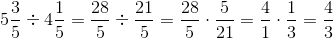### Example Question #3 : How To Divide Fractions

Express the quotient as a fraction in lowest terms: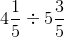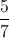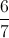Explanation:

Rewrite the mixed fractions as improper fractions, change to a multiplication by inverting the second, cross-cancel, and multiply across: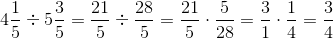### Example Question #4 : How To Divide Fractions

Evaluate: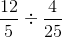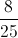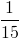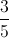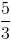Explanation:

Multiply by the reciprocal, cross-cancel, then multiply numerators and denominators: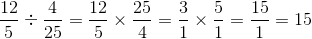### Example Question #5 : How To Divide Fractions

Evaluate: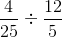Explanation:

Multiply by the reciprocal, cross-cancel, then multiply numerators and denominators: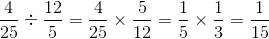### Example Question #6 : How To Divide Fractions

Evaluate: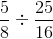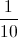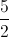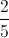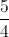Explanation:

Multiply by the reciprocal, cross-cancel, then multiply numerators and denominators: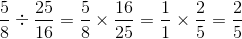### Example Question #7 : How To Divide Fractions

Evaluate: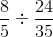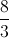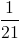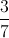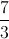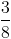Explanation:

Multiply by the reciprocal, cross-cancel, then multiply numerators and denominators: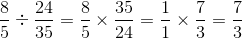### Example Question #8 : How To Divide Fractions

Evaluate: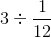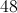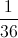Explanation: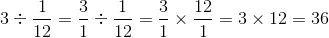### Example Question #9 : How To Divide Fractions

Evaluate: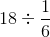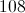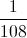Explanation: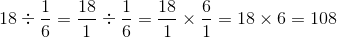### Example Question #10 : How To Divide Fractions

Evaluate: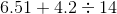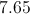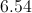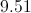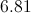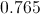Explanation:

By order of operations, divide first. Since the divisor is a whole number, divide as is: